Question 1
 Don’t just sit there! Build something!!

Learning to mathematically analyze circuits requires much study and practice. Typically, students practice by working through lots of sample problems and checking their answers against those provided by the textbook or the instructor. While this is good, there is a much better way.

You will learn much more by actually building and analyzing real circuits, letting your test equipment provide the “answers” instead of a book or another person. For successful circuit-building exercises, follow these steps:

1. Carefully measure and record all component values prior to circuit construction, choosing resistor values high enough to make damage to any active components unlikely.
2. Draw the schematic diagram for the circuit to be analyzed.
3. Carefully build this circuit on a breadboard or other convenient medium.
4. Check the accuracy of the circuit’s construction, following each wire to each connection point, and verifying these elements one-by-one on the diagram.
5. Mathematically analyze the circuit, solving for all voltage and current values.
6. Carefully measure all voltages and currents, to verify the accuracy of your analysis.
7. If there are any substantial errors (greater than a few percent), carefully check your circuit’s construction against the diagram, then carefully re-calculate the values and re-measure.

When students are first learning about semiconductor devices, and are most likely to damage them by making improper connections in their circuits, I recommend they experiment with large, high-wattage components (1N4001 rectifying diodes, TO-220 or TO-3 case power transistors, etc.), and using dry-cell battery power sources rather than a benchtop power supply. This decreases the likelihood of component damage.

As usual, avoid very high and very low resistor values, to avoid measurement errors caused by meter “loading” (on the high end) and to avoid transistor burnout (on the low end). I recommend resistors between 1 kΩ and 100 kΩ.

One way you can save time and reduce the possibility of error is to begin with a very simple circuit and incrementally add components to increase its complexity after each analysis, rather than building a whole new circuit for each practice problem. Another time-saving technique is to re-use the same components in a variety of different circuit configurations. This way, you won’t have to measure any component’s value more than once.

Question 2

In this circuit, a series resistor-capacitor network creates a phase-shifted voltage for the “gate” terminal of a power-control device known as a TRIAC. All portions of the circuit except for the RC network are “shaded” for de-emphasis: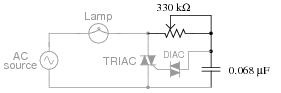Calculate how many degrees of phase shift the capacitor’s voltage is, compared to the total voltage across the series RC network, assuming a frequency of 60 Hz, and a 50% potentiometer setting.

Question 3

The following schematic diagram shows a simple crowbar circuit used to protect a sensitive DC load from accidental overvoltages in the supply power (+V):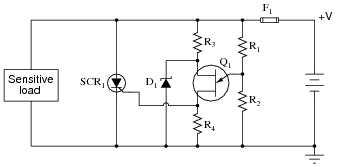Here, the UJT serves as an overvoltage detection device, triggering the SCR when necessary. Explain how this circuit works, and what the function of each of its components is.

Question 4

The circuit shown here indicates which pushbutton switch has been actuated first. After actuating any one of the three pushbutton switches (and energizing its respective lamp), none of the other lamps can be made to energize:Explain how this circuit works. Why can’t any of the other lamps turn on once any one of them has been energized? Also, explain how the circuit could be modified so as to provide a “reset” to turn all lamps off again.

Question 5

This crowbar circuit has a problem. It used to work just fine, and then one day it blew the fuse. Upon replacing the fuse, the new fuse immediately blew:Measuring the supply voltage with a voltmeter, everything checks out well. There does not appear to be an overvoltage condition causing a legitimate “crowbar” event in the circuit. Disconnecting the load from the crowbar circuit and powering it up with a standard bench-top laboratory power supply reveals the load to be in perfect condition. Thus, both the source and the load have been eliminated as possibilities that may have blown the fuse(s).

Moving on to the crowbar circuit itself, identify some component faults that could (each, independently) account for the problem, and explain your reasoning.

Question 6

What purpose does the TRIAC serve in this circuit?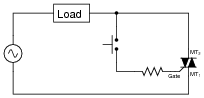Why use a TRIAC at all? Why not just use the switch to directly handle load current as in this next circuit?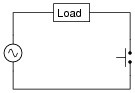Question 7

Optically-isolated TRIACs are available for use as solid-state relays, suitable for replacing electromechanical relays in many AC power switching applications: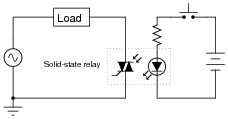Describe some of the advantages of using a solid-state relay for switching AC power instead of using an electromechanical relay as shown here: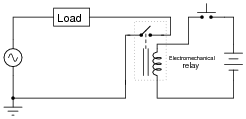Also describe any disadvantages to using a solid-state relay, if they exist.

Question 8

Predict how the operation of this AC power control circuit will be affected as a result of the following faults. Consider each fault independently (i.e. one at a time, no multiple faults):Switch contacts fail open:
Switch contacts fail shorted:
Resistor R1 fails open:
Solder bridge (short) past resistor R1:
Battery (V1) dies:

For each of these conditions, explain why the resulting effects will occur.

Question 9

This TRIAC circuit has a serious problem. Whenever the pushbutton switch is actuated, the TRIAC explodes!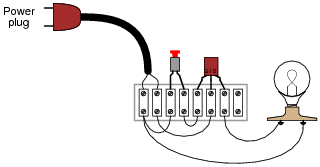Explain why this happens, and what must be done to fix the problem.

Question 10

Suppose a student builds the following TRIAC circuit and finds that it does not work: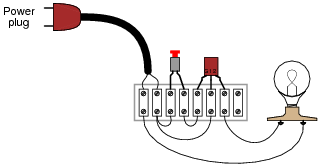When the pushbutton switch is actuated, nothing happens. What is wrong with this circuit? Hint: the problem in this circuit is very subtle, and may be very difficult to discern.

Question 11

A student builds this simple TRIAC power control circuit to dim a light bulb: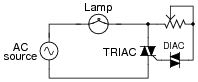The only problem with it is the lack of full control over the light bulb’s brightness. At one extreme of the potentiometer’s range, the light bulb is at full brightness. As the potentiometer is moved toward the direction of dimming, though, the light bulb approaches a medium level of intensity, then suddenly de-energizes completely. In other words, this circuit is incapable of providing fine control of power from “off” to “full” light. The range of control seems to be from full brightness to half-brightness, and nothing below that.

Connecting an oscilloscope across the light bulb terminals (using both channels of the oscilloscope to measure voltage drop in the “differential” mode), the waveform looks like this at full power: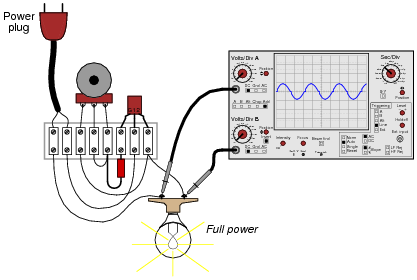When the potentiometer is adjusted to the position giving minimum light bulb brightness (just before the light bulb completely turns off), the waveform looks like this: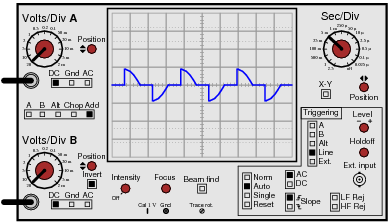Explain why this circuit cannot provide continuous adjustment of light bulb brightness below this level.

Question 12

Predict how the operation of this AC lamp dimmer circuit will be affected as a result of the following faults. Consider each fault independently (i.e. one at a time, no multiple faults):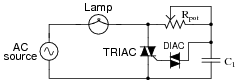Potentiometer Rpot fails open:
Capacitor C1 fails shorted:
Capacitor C1 fails open:
DIAC fails open:
TRIAC fails shorted:

For each of these conditions, explain why the resulting effects will occur.

Question 13

Explain how this battery charger circuit uses a TRIAC to control DC power to the battery:Also, identify some component failures in this circuit that could prevent DC power from getting to the battery.

Question 14

Commutation is an important issue in any kind of thyristor circuit, due to the “latching” nature of these devices. Explain what “commutation” means, and how it may be achieved for various thyristors.

Question 15

The following circuit exhibits very interesting behavior:When the power is first turned on, neither lamp will energize. If either pushbutton switch is then momentarily actuated, the lamp controlled by that SCR will energize. If, after one of the lamps has been energized, the other pushbutton switch is then actuated, its lamp will energize and the other lamp will de-energize.

Stated simply, each pushbutton switch not only serves to energize its respective lamp, but it also serves to de-energize the other lamp as well. Explain how this is possible. It should be no mystery to you why each switch turns on its respective lamp, but how is the other switch able to exert control over the other SCR, to turn it off?

Hint: the secret is in the capacitor, connected between the two SCRs’ anode terminals.

Question 16

The following schematic diagram shows a timer circuit made from a UJT and an SCR:Together, the combination of R1, C1, R2, R3, and Q1 form a relaxation oscillator, which outputs a square wave signal. Explain how a square wave oscillation is able to perform a simple time-delay for the load, where the load energizes a certain time after the toggle switch is closed. Also explain the purpose of the RC network formed by C2 and R4.

Related Tools:

Published under the terms and conditions of the Creative Commons Attribution License